SLVSD43C May   2015  – February 2019

PRODUCTION DATA.

1. Features
2. Applications
3. Description
4. Revision History
5. Pin Configuration and Functions
6. Specifications
7. Detailed Description
1. 7.1 Overview
2. 7.2 Functional Block Diagram
3. 7.3 Feature Description
4. 7.4 Device Functional Modes
8. Application and Implementation
1. 8.1 Application Information
2. 8.2 Typical Application
1. 8.2.1 Design Requirements
2. 8.2.2 Detailed Design Procedure
3. 8.2.3 Application Curves
9. Power Supply Recommendations
10. 10Layout
11. 11Device and Documentation Support
12. 12Mechanical, Packaging, and Orderable Information

• PWP|28
• PWP|28

### 6.7 Typical Characteristics

VIN = 14 V, VEN ≥ 2 V, TJ = –40°C to +150°C (unless otherwise noted)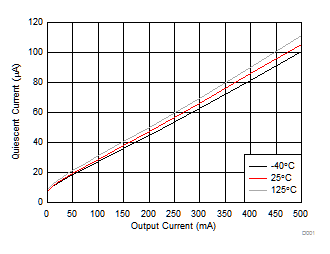Figure 1. Quiescent Current vs Output Current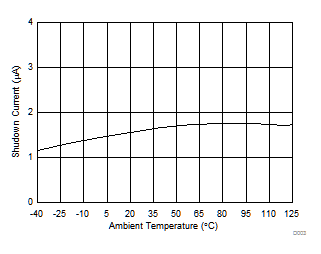Figure 3. Shutdown Current vs Ambient Temperature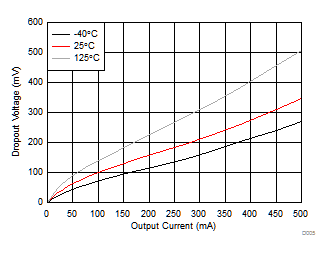Figure 5. Dropout Voltage vs Output Current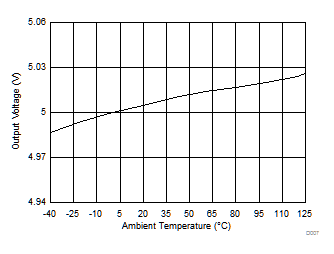VOUT = 5 V
Figure 7. Output Voltage vs Ambient TemperatureVIN = 5.6 V
Figure 9. Output Current Limit (ILIM) vs Ambient Temperature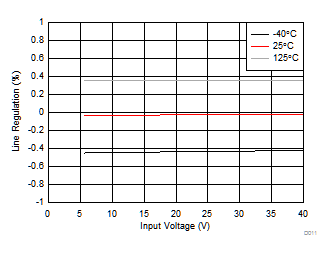Figure 11. Line Regulation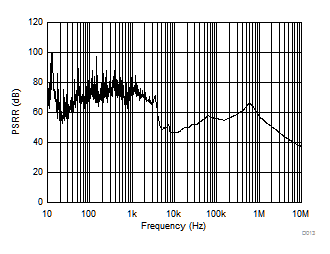COUT = 10 μF IOUT = 100 mA TA = 25°C
Figure 13. PSRR vs Frequency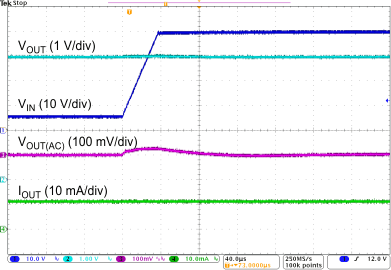VIN = 6 V to 40 V VOUT = 5 V COUT = 10 µF IOUT = 1 mA
Figure 15. Line Transient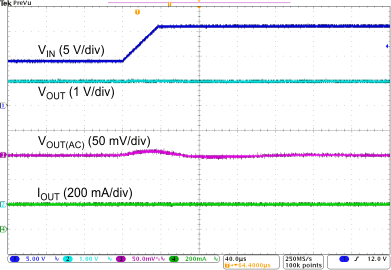VIN = 6 V to 40 V VOUT = 5 V COUT = 10 µF IOUT = 200 mA
Figure 17. Line Transient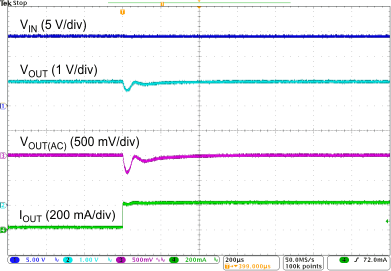VOUT = 5 V COUT = 10 µF IOUT = 1 mA to 200 mA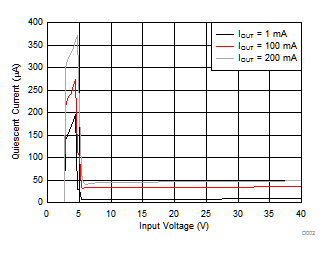Figure 2. Quiescent Current vs Input Voltage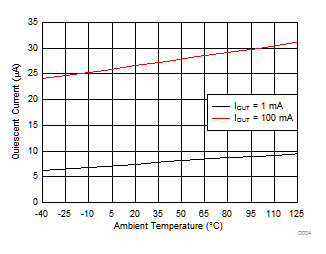Figure 4. Quiescent Current vs Ambient Temperature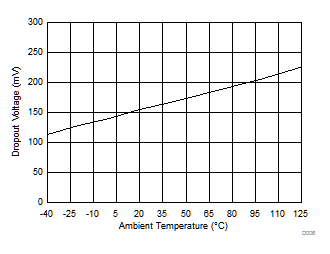IOUT = 200 mA
Figure 6. Dropout Voltage vs Ambient Temperature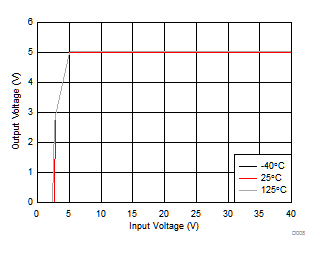VOUT = 5 V
Figure 8. Output Voltage vs Input Voltage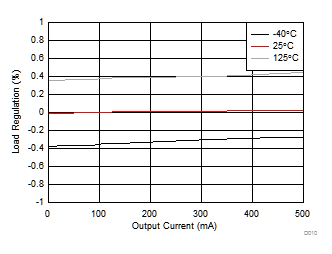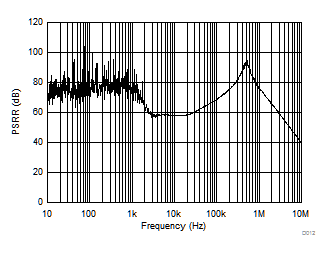COUT = 10 μF IOUT = 1 mA TA = 25°C
Figure 12. PSRR vs Frequency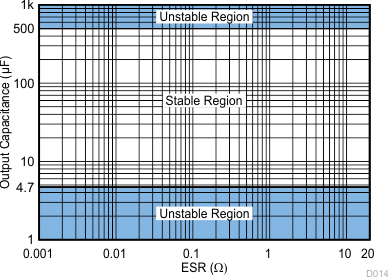Figure 14. ESR Stability vs Output Capacitance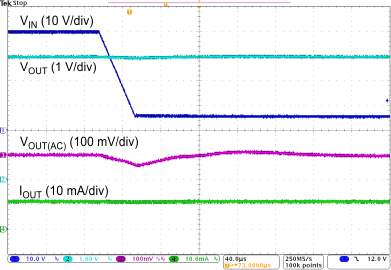VIN = 40 V to 6 V VOUT = 5 V COUT = 10 µF IOUT = 1 mA
Figure 16. Line Transient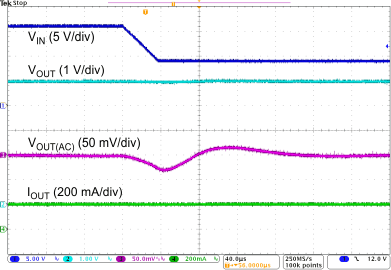VIN = 40 V to 6 V VOUT = 5 V COUT = 10 µF IOUT = 200 mA
Figure 18. Line Transient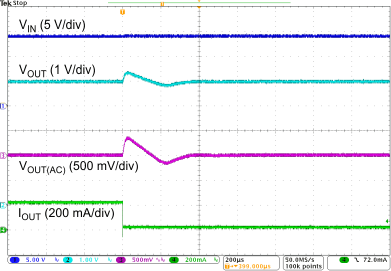VOUT = 5 V COUT = 10 µF IOUT = 200 mA to 1 mA